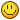## Recommended Posts

Hey guys,

I'm having an odd issue. I'm trying to automatically generate a map. Here, I'm going to show the code, and then explain what happens when I run it:

`    // This is another class and defines a room in the game    Room: function(x, y, w, h) {        this.x1 = x;        this.y1 = y;        this.x2 = x + w;        this.y2 = y + h;            },    // Used to create rooms (a rectangle of non-blocked tiles) on the map    // SOMETHING HERE IS CRASHING     createRoom: function(room) {        for (var x = room.x1; x < room.x2; x=+32) {            for (var y = room.y1; y < room.y2; y=+32){                // change the "true" to "false" after collision is worked out                this.map.push(new this.Tile(x, y, true, true));            }        }    },`

(I'm sorry, I know this code isn't pretty)

Okay, so, as you can see, a room is really just a coordinate-defined rectangle. The "map" is an array that stores "Tiles" which are coordinate-defined tiles. Whew, does that make sense? I need to go back to school for sure.

Now, that for statement, I'm going to use an example: We'll assume that room.x1 = 32, ".x2 = 96, ".y1 = 32, ".y2 =96. Nice and easy.

(1) In the first for statement: if 32  is less than 96; it is, so we change 32 to 64 and move on

(2) In the 2nd for statement: if 32  is less than 96; it is, so we change 32 to 64 and move on

(3) We append a tile to the map array with coords  (64, 64), and go back to the beginning

(5) In the next pass we append a tile with coords (96, 96), and go back to the beginning

(6) The for statement no longer applies, so it stops.

This should only run 3 times right? But it's running indefinitely and crashing my browser.

Any ideas?

##### Share on other sites

You've got 2 infinite loops due to typos on lines 13 and 14. Instead of incrementing by 32 with "+=", you are setting the loop variable with "=" to "+32"

##### Share on other sites

Yeah, chg is right, modifying the existing value is done by putting the operator before the equals sign, so += will increment the var on the left by the amount on the right, *= will multiply, /= will divide and so on.

##### Share on other sites

That will fix your infinite loop but, your description of what you expect that loop to do is wrong. It will push 4 tiles into the array with (x, y) in order (32, 32), (32, 64), (64, 32), (64, 64). If you want it to include 96, change '<' into '<='.

The way you describe this "In the first for statement: if 32  is less than 96; it is, so we change 32 to 64 and move on" makes it seem like you do not understand the order in which for loops work.

`for (a; b; c) {  d}`

a is executed before the loop d starts.

b defines the condition for running the loop and is checked before every iteration.

c is executed each time after the loop d has been executed.

##### Share on other sites

Omfg! Well, If you can't tell, I'm "self-taught" (unfortunately classes in programming were not provided for a degree in Network and Server Administration). I thank you all for your answers, and I apologize for bugging with something so obvious.## Create an account or sign in to comment

You need to be a member in order to leave a comment

## Create an account

Sign up for a new account in our community. It's easy!

Register a new account